# KSEEB Solutions for Class 7 Maths Chapter 1 Integers Ex 1.4

Students can Download Chapter 1 Integers Ex 1.4, Question and Answers, Notes Pdf, KSEEB Solutions for Class 7 Maths, Karnataka State Board Solutions help you to revise complete Syllabus and score more marks in your examinations.

## Karnataka State Syllabus Class 7 Maths Chapter 1 Integers Ex 1.4

Question 1.
Evaluate each of the following
a) (-30) ÷ 10
Solution: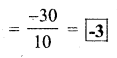b) 50 ÷ (-5)
Solution: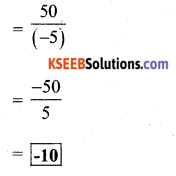c) (-36) ÷ (-9)
Solution: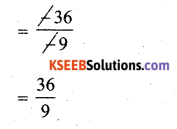= 4

d) (-49) ÷ (49)
Solution: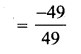= -1

e) 13 ÷ [(-2) + 1]
Solution:
= 13 ÷ (-2 + 10)
= 13 ÷ (-1)
$$=\frac{13}{-1}$$
= -13f) 0 ÷ (-12)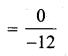= 0

g) (-31) ÷ [(-30) + (-1)]
= (-31) ÷ [-30 – 1]
= (-31) ÷ (-31)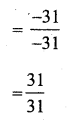= 1

h) [(-36) ÷ 12] ÷ 3
Solution: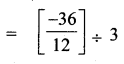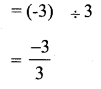= -1

i) [(-6) + 5] ÷ [(- 2) + 1]
=[-6 + 5] ÷ [-2 + 1]
= (-1) ÷ (-1)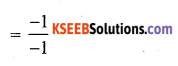= 1

Question 2.
Verify that a ÷ (b + c) ≠ (a ÷ b) + (a ÷ c) for each of the following values of a, b and c.
a) a = 12, b = -4, c = 2
Solution:
a) a = 12, b = -4, c = 2
To verify a ÷ (b + c) ≠ (a ÷ b) + (a ÷ c)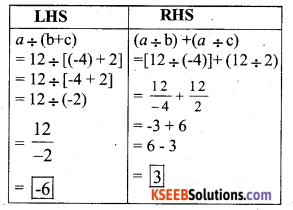∴ LHS ≠ RHS
hence verifiedb) a = (-10), b = 1, c = 1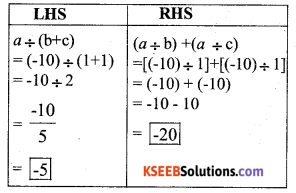∴ LHS ≠ RHS
hence verified

Question 3.
Fill in the blanks :

a) 369 ÷ ___ = 369
369 ÷ 1 = 369
Let number be x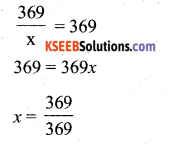x = 1

b) (-75) ÷ ___ = -1
(-75) ÷ 75 = -1
Let number be x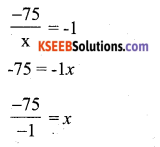x = 75

c) (-206) ÷ ___ = 1
(-206) ÷ -206 = 1
Let number be x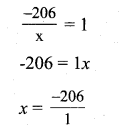x = -206

d) -87 ÷ ___ = 87
-87 ÷ -1 = 87
let the number be x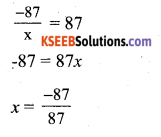x = -1e) ___ ÷ 1 = -87
87 ÷ 1 = -87
Let the number be x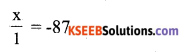1 × -87 = x
-87 = x
x = -87

f) ___ ÷ 48 = -1
48 ÷ 48 = -1
Let number be x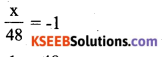– 1 × 48 = x
-48 = x
∴ x = -48

g) 20 ÷ ___ = -2
20 ÷ -10 = (-2)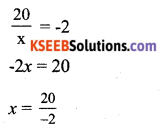x = -10h) __ ÷ (4) = -3
-12 ÷ (4) = -3
Let number be x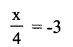x = -3 × 4
x=-12

Question 4.
Write five pairs of integers (a, b) such that a – b = -3. One such pair is (6, -2) because 6 ÷ (-2) = (-3)
Solution:
a ÷ b = -3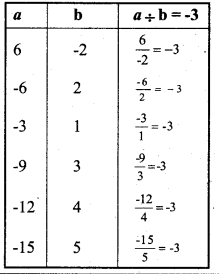∴ The other five pairs are (-6, 2), (-3, 1), (-9, 3), (-12, 4), (-15, 5)

Question 5.
The temperature at 12 noon was 10°C above zero. If it decreases at the rate of 2°C per hour until midnight, at what time would the temperature be 8°C below zero? What would be the temperature at mid-night ?
Solution:
Temperature at 12pm = 10°C
Temperature decreases at 2°C per hour
we need to find, time when temperature is 8°C below zero, ie., -8°C
∴ Total decrease = Final temperature – initial temperature = -8°C -10°C
= -8 – 10 = -18°C
Now,
Temperature decrease in 1 hour × Total number of hours = Total decrease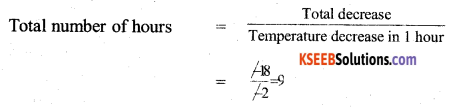So, temperature becomes -8°C = 9 hours
∴ Temperature is -8°C at 9PM
Now,
We need to find temperature at mid – night temperature at 12AM = Temperature at 12PM
Temperature change in 12 hours
Temperature change in 1 hour = -2°C
Temperature change in 12 hour = -2 × 12°C = -24°C
Now,
Temperature at 12AM – Temperature at 12PM = Temperature change in 12 hours. Temperature at 12AM – 10°C = -24°C
Temperature at 12AM = – 24°C + 10°C
Temperature at 12 AM = -14°C
∴ Temperature at Mid – night = -14°CQuestion 6.
In a class test (+3) marks are given for every correct answer and (-2) marks are given for every incorrect answer and no marks for not attempting any question.
Solution:
Marks given for correct answer = +3
Marks given for incorrect answer = -2
Marks given for not attempting = 0
i) Radhika scored 20 marks. If she has got 12 correct answers, how many questions has she attempted incorrectly ?
Solution:
Total marks scored = 20
Number of correct answer = 12
Marks of for correct answer = 12 × 3 = 36
Also
Marks for not attempt answer = 0 × Number of not attempt = 0
Now,
Total marks = marks for correct answer + marks for incorrect answer + marks for not attempt
20 = 36 + (-2) × No of incorrect answers + 0
20 – 36 = (-2) × No of incorrect answers
-16 = (-2) × No of incorrect answer
$$\frac{-16}{-2}$$ = No of incorrect answers
8 = No of incorrect answers
So, Radhika attempted 8 questions incorrectly

ii) Mohini scores -5 marks in this test, thought she has got 7 correct answers. How many questions has she attempted incorrectly?
Solution:
Total marks = – 5
No of correct answers = 7
Marks for correct answers = 7 × 3 = 21
Also,
Marks for incorrect answer = (- 2) × No. of incorrect answers
Marks for not attempt answer = 0 × No of not attempt answers
Now,
Total marks = marks for correct answer + marks for incorrect answer + marks for not attempt
– 5 = 21 + (- 2) × No. of incorrect answers + 0
– 5 – 21 = (- 2) × No. of incorrect answers
– 26 = – 2 × No. of incorrect answers
$$\frac{-26}{-2}$$ = No. of incorrect answers
13 = No, of incorrect answers.
∴ Mohini attempted 13 questions incorrectlyQuestion 7.
An elevator descends into a mine shaft at the rate of 6m/min. If the descent starts from 10m above the ground level, how long will it take to reach -350 m.
Solution:
Initial position = 10m final position = -350 m
Total distance elevator has to travel = initial position – final position
= 10 – (- 350)
= 10 + 350 = 360 m
Now,
Elevator descends at rate 6m/min
∴ Elevator travels 6m in = 1 minute
Elevator travels 1 m in: = $$\frac{1}{6}$$
Elevator travels 360m in = $$\frac{1}{6}$$ × 360 minutes
= 60 minutes
= 1 hour
∴ It will take the elevator 1 hour.